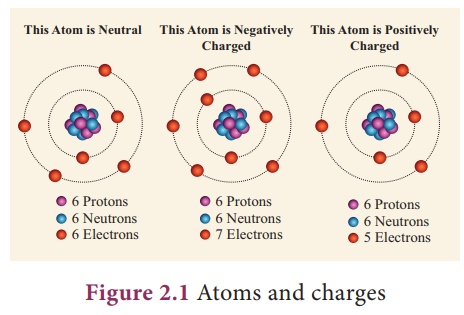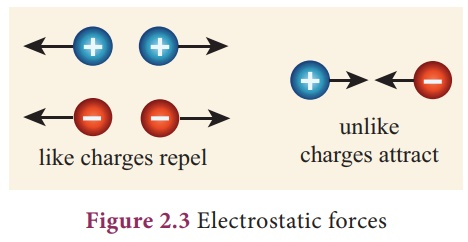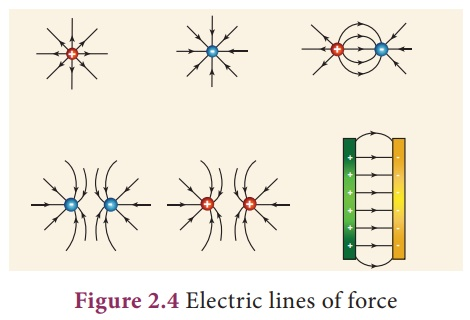Home | | Science 9th std | Electric charges

# Electric charges

As electrons are revolving in the orbits of an atom, they can be easily removed from an atom and also added to it. If an electron is removed from the atom, the atom becomes positively charged.

Electric charges

We know that all matter is made up of tiny particles called, atoms. Inside each atom there is a nucleus with positively charged protons and chargeless neutrons and negatively charged electrons orbiting the nucleus. Usually there are as many electrons as there are protons and the atoms themselves are neutral.

As electrons are revolving in the orbits of an atom, they can be easily removed from an atom and also added to it. If an electron is removed from the atom, the atom becomes positively charged. Then it becomes a positive ion. If an electron is added in excess to an atom then the atom is negatively charged. This atom is called negative ion. More than one electron can also be removed from or added to atoms to make them accordingly more positive and more negative (Fig. 2.1).When you rub a plastic comb on your dry hair, the comb obtains power to attract small pieces of paper, is it not? When you rub the comb vigorously, electrons from your hair leave and accumulate on the edge of the comb. Your hair is now positively charged as it has lost electrons. The comb is negatively charged as it has gained electrons (Fig. 2.2).## 1. Measuring electric charge

Electric charge is measured in coulomb and the symbol for the same is C. The charge of an electron is numerically a very tiny value. The charge of an electron (represented as e) is the fundamental unit with a charge equal to 1.6 x 10-19 C. This indicates that any charge (q) has to be an integral multiple (n) of this fundamental unit of electron charge (e).

q = ne

here, n is a whole number.

Can you guess how many electrons accumulate to make 1 C of electric charge?

### Solution:

Charge on 1 electron, e = 1.6 x 10–19 C

q=ne or n=q/e

number of electrons in 1 coulomb= 6.25 x 1018 electrons

Practically, we also have µC (micro coulomb) nC (nano coulomb)and pC (pico coulomb) as units of electric charge.

1 µC = 10-6 C, 1nC=10-9 and 1pC = 10-12C

Electric charge is additive in nature. e total electric charge of a system is the algebraic sum of all the charges located in the system. For example, let us say a system has two charges +5C and –2C. en the total or net charge on the system is, (+5C) + (–2C) = +3C.

## 2. Electric force

Among electric charges there are two types of electric force (F). One is attractive and another is repulsive. The like charges repel and unlike charges attract. The force existing between the charges is called as ‘electric force’. These forces are non-contact forces, and hence can be experienced even when the charges are not in contact.The numerical value (magnitude) of electric force between two charges depend on the,

i.               value of charges on them,

ii.               distance between them and

iii.               nature of medium between them.

## 3. Electric field

The region in which a charge experiences electric force forms the ‘electric field’ around the charge. O en electric field (E) is represented by lines and arrowheads indicating the direction of the electric led (Fig. 2.4). The direction of the electric field is the direction of the force that would act on a small positive charge. erefore the lines representing the electric field are called ‘electric lines of force’. The electric lines of force are straight or curved paths along which a unit positive charge tends to move in the electric field. Electric lines of force are imaginary lines. The strength of an electric field is represented by how close the field lines are to one another.

For an isolated positive charge the electric lines of force are radially outwards and for an isolated negative charge they are radially inwards.Electric field at a point is a measure of force acting on a unit positive charge placed at that point. A positive charge will experience force in the direction of electric field and a negative charge will experience in the opposite direction of electric field.

## 4. Electric potential

Though there is an electric force (either attractive or repulsive) existing among the charges, they are still kept together, is it not?. We now know that in the region of electric charge there is an electric field. Other charges experience force in this field and vice versa. ere is a work done on the charges to keep them together. is results in a quantity called ‘electric potential’.Electric potential is a measure of the work done on unit positive charge to bring it to that point against all electrical forces. The electric potential (V) near positive charges is positive and near a negative charges is negative. Other positive charges have a tendency to move from higher potential to lower potential and negative charges the other way.

Study Material, Lecturing Notes, Assignment, Reference, Wiki description explanation, brief detail
9th Science : Electric charge and electric current : Electric charges |